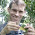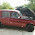## Thursday, March 28, 2013

### Marginal ancestral state reconstruction using multiple methods

"A quick question to follow up on your two posts, and some recent discussion on the R list: do you have a good feeling for the quickest implementation to do this on large trees? (Happy to assume for the purposes of this question that I'm O.K. with the stochastic variation in stochastic character mapping, and that I'm not worried about the differences between marginal frequencies and marginal probabilities...)"

I was about to submit a response to the comment directly - but then it occurred to me that other readers might be interested as well.

The fastest in R seems to be ASR using Rich Fitzjohn's package diversitree, although it is a little more difficult to use than what I have in phytools.

I did not compare, however, to phangorn, which Klaus Schliep showed us can also be used quite nicely.

Here's some demo code, including simulation, with comments:

require(phytools)
require(diversitree)

# simulate using phytools:
tree<-pbtree(n=100,scale=1)
Q<-matrix(c(-2,1,1,1,-2,1,1,1,-2),3,3)
rownames(Q)<-colnames(Q)<-1:3
x<-sim.history(tree,Q)\$states

# ASR using rerootingMethod
XX<-rerootingMethod(tree,x,model="ER")
# ASRs in XX\$marginal.anc

# ASR using make.simmap
mtrees<-make.simmap(tree,x,model="ER",nsim=100)
# (we should ideally increase nsim, if possible)
YY<-describe.simmap(mtrees)
# ASRs in YY\$ace

# ASR using diversitree
y<-setNames(as.numeric(x),names(x))
# (we needed to convert to numeric)
lik<-make.mkn(tree,y,k=3)
# constrain to ER model
lik<-constrain(q13~q12,q21~q12,q23~q12,q31~q12,q32~q12)
fit<-find.mle(lik,setNames(1,argnames(lik)))
ZZ<-t(asr.marginal(lik,coef(fit)))
dimnames(ZZ)<-dimnames(XX\$marginal.anc)

(**Note that I'm not that experienced with diversitree, so please post corrections if the above was not done properly.)

In theory, rerootingMethod & asr.marginal should give identical estimates for any model in which all qij=qji. make.simmap will give different ancestral state estimates, but these will be highly correlated as nsim is made to be large.

1.Realized in trying to repeat this that:

lik<-constrain(q13~q12,q21~q12,q23~q12,q31~q12,q32~q12)

should be:

lik<-constrain(lik,q13~q12,q21~q12,q23~q12,q31~q12,q32~q12)

2.Hi
I'm running several R packages to ancestral state reconstruction on discrete data and your blog is a great help to me.
I have a question about diversitree package.
I'm testing diversitree package and i don't find examples to implement ARD model on diversitree.
Do you think my syntax is correct?

lik <- make.mk2(mytree_ultra, vect, strict=FALSE)
fit <- find.mle(lik,setNames(c(1,2),argnames(lik))
asr <- asr.marginal(lik,coef(fit))

Thanks

Note: due to the very large amount of spam, all comments are now automatically submitted for moderation.